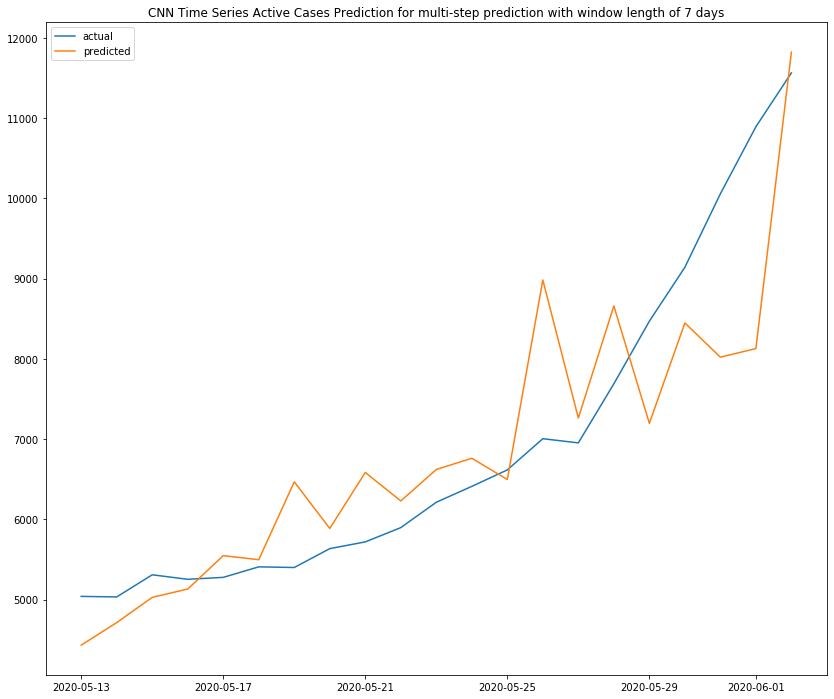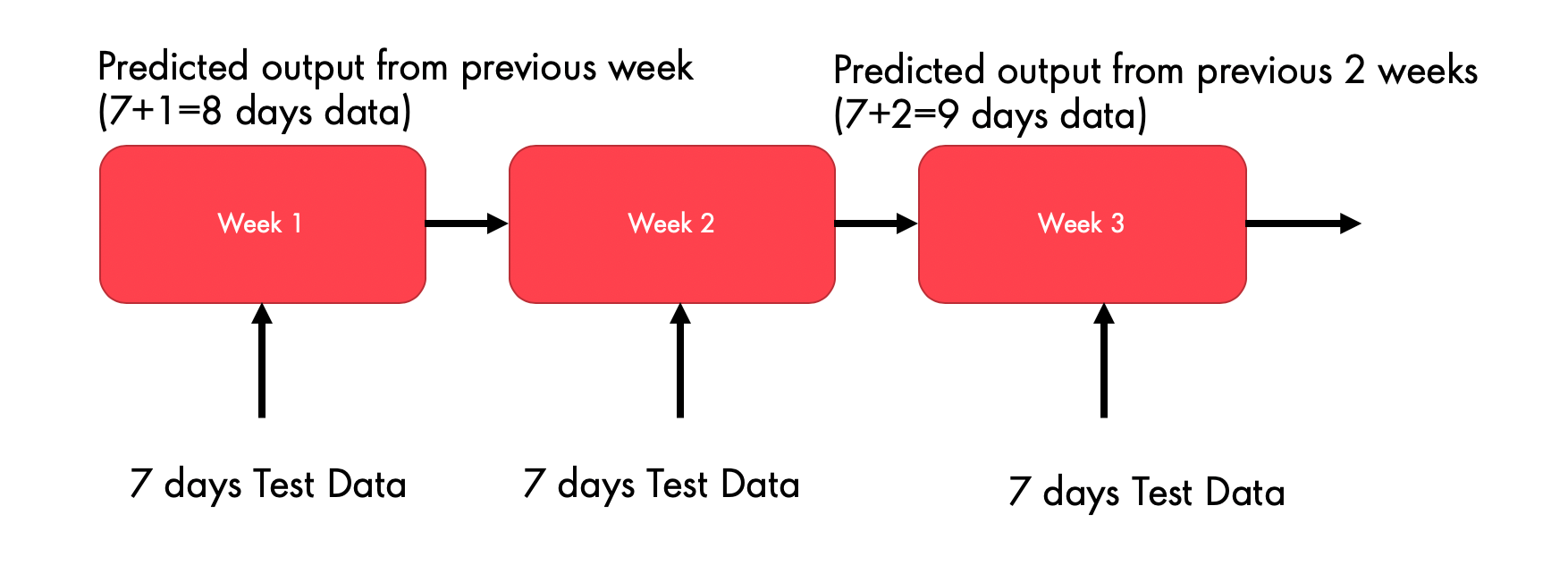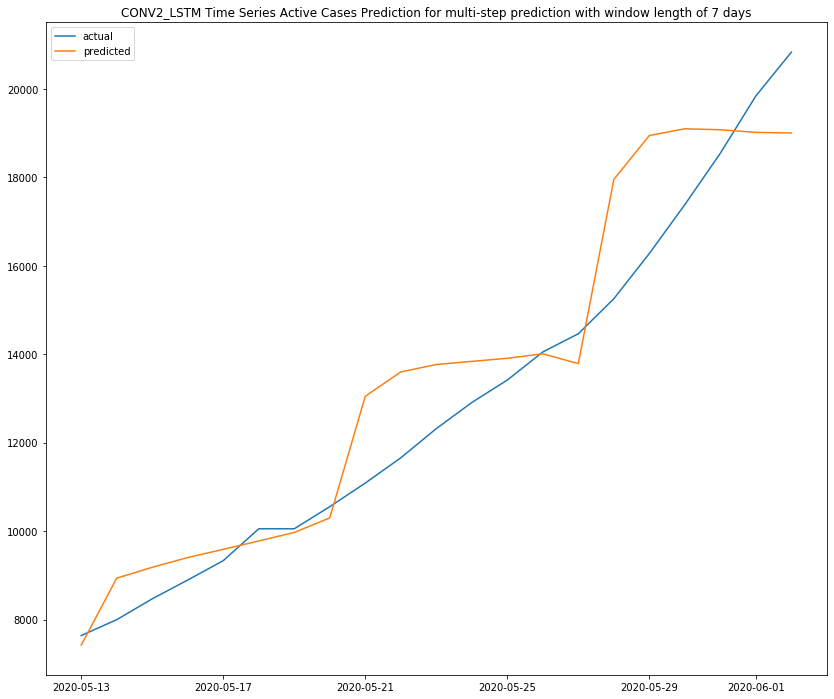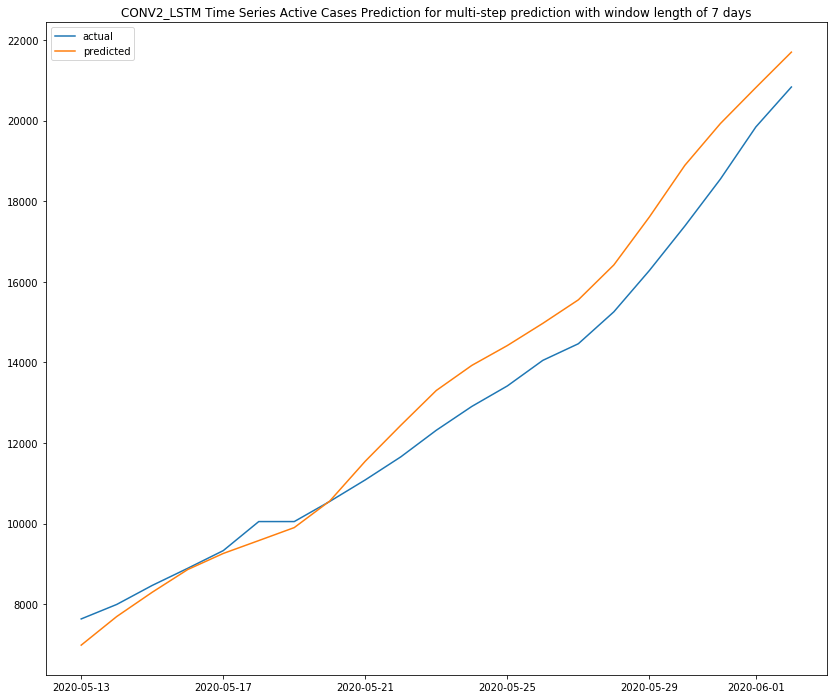# Genius Tool to Compare Best Time-Series Models For Multi-step Time Series Modeling from Featured Blog Posts – Data Science Central

0
486Predict Number of Active Cases by Covid-19 Pandemic based on Medical Facilities (Volume of Testing, ICU beds, Ventilators, Isolation Units, etc) using Multi-variate LSTM based Multi-Step Forecasting Models

## Introduction and Motivation

The intensity of the growth of the covid-19 pandemic worldwide has propelled researchers to evaluate the best machine learning model that could the people affected in the distant future by considering the current statistics and predicting the near future terms in subsequent stages.

While different univariate models like ARIMA/SARIMA and traditional time-series are capable of predicting Number of Active cases, daily recoveries, Number of deaths, they do not take into consideration the other time-varying factors like Medical Facilities (Volume of Testing, ICU beds, Hospital Admissions, Ventilators, Isolation Units, Quarantine Centres, etc).As these factors become important we build a predictive model that can predict the Number of Active Cases, Deaths, and Recoveries based on the change in Medical Facilities as well as other changes in infrastructure.

Here in this blog, we try to model Multi-step Time Series Prediction using Deep learning Models on the basis of Medical Information available for different states of India.

## Multi-Step Time Series Prediction

A typical multi-step predictive model looks as the below figure, where each of the predicted outcomes from the previous state is treated as next state input to derive the outcome for the second-state and so forth.www.tensorflow.org/tutorials/structured_data/time_series_files/output_0xJoIP6PMWMI_1.png?resize=429%2C280&ssl=1” alt=”png” width=”429″ height=”280″ /> Source

## Deep Learning-based Multi-variate Time Series Training and Prediction

The following figure illustrated the important steps involved in selecting the best deep learning model.Time-Series based Single/Multi-Step Prediction
• Feeding Multi-variate data from a single source or from aggregated sources available directly from the cloud or other 3rd-party providers into the ML modeling data ingestion system.
• Cleaning, preprocessing, and feature engineering of the data involving scaling and normalization.
• Conversion of the data to a supervised time-series.
• Feeding the data to a deep learning training source that can train different time-series models like LSTM, CNN, BI-LSTM, CNN+LSTM using different combinations of hidden layers, neurons, batch-size, and other hyper-parameters.
• Forecasting based on near term or far distant term in future either using Single-Step or Multi-Step Forecasting respectively
• Evaluation of some of the error metrics like (MAPE, MAE, ME, RMSE, MPE) by comparing it with the actual data, when it comes in
• Re-training the model and continuous improvements when the threshold of error exceeds.

## Import Necessary Tensorflow Libraries

The code snippet gives an overview of the necessary libraries required for tensorflow.

``from tensorflow.python.keras.layers import Dense, LSTM, RepeatVector,TimeDistributed,Flatten, Bidirectional from tensorflow.python.keras import Sequential  from tensorflow.python.keras.layers.convolutional import Conv1D, Conv2D, MaxPooling1D,ConvLSTM2D``

As Delhi had high Covid-19 cases, here we model different DL models for the “DELHI” State (National Capital of India). Further, we keep the scope of dates from 25th March to 6th June 2020. Data till 29th April has been used for Training, whereas from 30th April to 6th June has been used for testing/prediction. The test data has been used to predict for 7 days for 3 subsequent stages of prediction.

This code demonstrates the data is first split into a 70:30 ratio between training and testing (by finding the closest number to 7), where each set is then restructured to weekly samples of data.

``def split_dataset(data): # split into standard weeks     print(np.shape(data))     split_factor = int((np.shape(data)*0.7))     print("Split Factor no is", split_factor)      m = 7     trn_close_no = closestNumber(split_factor, m)     te_close_no = closestNumber((np.shape(data)-split_factor), m)      train, test = data[0:trn_close_no], data[trn_close_no:(trn_close_no + te_close_no)]     print("Initials Train-Test Split --", np.shape(train), np.shape(test))      len_train = np.shape(train)     len_test = np.shape(test)      # restructure into windows of weekly data     train = array(split(train[0:len_train], len(train[0:len_train]) / 7))    test = array(split(test, len(test) / 7))      print("Final Train-Test Split --", np.shape(train), np.shape(test))     return train, test``
`Initials Train-Test Split -- (49, 23) (21, 23) ----- Training and Test DataSet Final Train-Test Split -- (7, 7, 23) (3, 7, 23) ----- Arrange Train and Test DataSet into 7 and 3 weekly samples respecytively.`

The data set and the features have been scaled using Min-Max Scaler.

``scaler = MinMaxScaler(feature_range=(0, 1)) scaled_dataset = scaler.fit_transform(dataset)``

## Convert Time-Series to a Supervised DataSet

The tricky part in converting the time-series to a supervised time-series for multi-step prediction lies in incorporating the number of past days (i.e. the historic data) that the weekly data has to consider.

The series derived by considering historic data is considered 7 times during training iterations and 3 times during testing iterations (as it got split as (7,7,23) and (7,3,23), where 22 is the number of input features with one predicted output). This series built using historic data helps the model to learn and predict any day of the week.

#### Note 1: This is the most important step of formulating a time-series data to a multi-step model

The below snippet code demonstrates what is described above.

``# convert history into inputs and outputs def to_supervised(train, n_input, n_out=7):     # flatten data     data = train.reshape((train.shape * train.shape, train.shape))     X, y = list(), list()     in_start = 0     # step over the entire history one time step at a time     for _ in range(len(data)):        # define the end of the input sequence         in_end = in_start + n_input         out_end = in_end + n_out         # ensure we have enough data for this instance         if out_end <= len(data):             X.append(data[in_start:in_end, :])             y.append(data[in_end:out_end, 0])         # move along one time step         in_start += 1     return array(X), array(y)``

## Training Different Deep Learning Models using Tensorflow

In this section, we describe how we train different DL models using Tensorflow’s Keras APIs.

#### Convolution Neural Network (CNN Model)

The following figure recollects the structure of a Convolution Neural Network (CNN) with a code snippet showing how a 1D CNN with 16 filters, with a kernel size of 3 has been used to train the network over 7 steps, where each 7 step is of 7 days.

``# train CNN model def build_model_cnn(train, n_input):     # prepare data     train_x, train_y = to_supervised(train, n_input)     # define parameters     verbose, epochs, batch_size = 0, 200, 4     n_timesteps, n_features, n_outputs = train_x.shape, train_x.shape, train_y.shape     # define model     model = Sequential()     model.add(Conv1D(filters=16, kernel_size=3, activation='relu', input_shape=(n_timesteps,n_features)))     model.add(MaxPooling1D(pool_size=2))model.add(Flatten())     model.add(Dense(10, activation='relu'))     model.add(Dense(n_outputs))     model.compile(loss='mse', optimizer='adam')     # fit network     model.fit(train_x, train_y, epochs=epochs, batch_size=batch_size, verbose=verbose)     return model``CNN

#### LSTM

The following code snippet demonstrates how we train an LSTM model, plot the training and validation loss, before making a prediction.

``# train LSTM model def build_model_lstm(train, n_input):          # prepare data     train_x, train_y = to_supervised(train, n_input)     print(np.shape(train_x))     print(np.shape(train_y))     # define parameters     verbose, epochs, batch_size = 0, 50, 16     n_timesteps, n_features, n_outputs = train_x.shape, train_x.shape, train_y.shape     # reshape output into [samples, timesteps, features]     train_y = train_y.reshape((train_y.shape, train_y.shape, 1))     # define model     model = Sequential()     model.add(LSTM(200, activation='relu', input_shape=(n_timesteps, n_features)))     model.add(RepeatVector(n_outputs))     model.add(LSTM(200, activation='relu', return_sequences=True))     model.add(TimeDistributed(Dense(100, activation='relu')))     model.add(TimeDistributed(Dense(1)))     model.compile(loss='mse', optimizer='adam')     # fit network     model.fit(train_x, train_y, epochs=epochs, batch_size=batch_size, verbose=verbose)     return model``

The below figure illustrates the Actual vs Predicted Outcome of the Multi-Step LSTM model after the predicted outcome has been inverse-transformed (to remove the effect of scaling).LSTM

#### Bi-Directional LSTM

The following code snippet demonstrates how we train a BI-LSTM model, plot the training and validation loss, before making a prediction.Source
``# train Bi-Directionsl LSTM model def build_model_bi_lstm(train, n_input):# prepare data     train_x, train_y = to_supervised(train, n_input)     print(np.shape(train_x))     print(np.shape(train_y))     # define parameters     verbose, epochs, batch_size = 0, 50, 16     n_timesteps, n_features, n_outputs = train_x.shape, train_x.shape, train_y.shape# reshape output into [samples, timesteps, features]     train_y = train_y.reshape((train_y.shape, train_y.shape, 1))     # define model     model = Sequential()     model.add(Bidirectional(LSTM(200, activation='relu', input_shape=(n_timesteps, n_features))))     model.add(RepeatVector(n_outputs))     model.add(Bidirectional(LSTM(200, activation='relu', return_sequences=True)))     model.add(TimeDistributed(Dense(100, activation='relu')))     model.add(TimeDistributed(Dense(1)))     model.compile(loss='mse', optimizer='adam')     # fit network     model.fit(train_x, train_y, epochs=epochs, batch_size=batch_size, verbose=verbose)     return model``

The below figure illustrates the Actual vs Predicted Outcome of Multi-Step Bi-LSTM model after the predicted outcome has been inverse-transformed (to remove the effect of scaling).BI-LSTM

#### Stacked LSTM + CNN

Here we have used Conv1d with TimeDistributed Layer, which is then fed to a single layer of LSTM, to predicted different sequences, as illustrated by the figure below. The CNN model is built first, then added to the LSTM model by wrapping the entire sequence of CNN layers in a TimeDistributed layer.Source
``# train Stacked CNN + LSTM model def build_model_cnn_lstm(train, n_input):     # prepare data     train_x, train_y = to_supervised(train, n_input)     # define parameters     verbose, epochs, batch_size = 0, 500, 16     n_timesteps, n_features, n_outputs = train_x.shape, train_x.shape, train_y.shape     # reshape output into [samples, timesteps, features]     train_y = train_y.reshape((train_y.shape, train_y.shape, 1))     # define model     model = Sequential()     model.add(Conv1D(filters=64, kernel_size=3, activation='relu', input_shape=(n_timesteps, n_features)))     model.add(Conv1D(filters=64, kernel_size=3, activation='relu'))     model.add(MaxPooling1D(pool_size=2))     model.add(Flatten())     model.add(RepeatVector(n_outputs))     model.add(LSTM(200, activation='relu', return_sequences=True))     model.add(TimeDistributed(Dense(100, activation='relu')))     model.add(TimeDistributed(Dense(1)))     model.compile(loss='mse', optimizer='adam')     # fit network     model.fit(train_x, train_y, epochs=epochs, batch_size=batch_size, verbose=verbose)     return model``

The prediction and inverse scaling help to yield the actual predicted outcomes, as illustrated below.LSTM With CNN

## Multi-Step Forecasting and Evaluation

The below snippet states how the data is properly reshaped into (1, n_input, n) to forecast for the following week. For the multi-variate time-series (of 23 features) with test data of 23 samples (with predicted output from previous steps i.e. 21+2) for 3 weeks is reshaped from (7,7,23), (8,7,23) and (9,7,23) as (49,23), (56,23) and (63, 23)Prediction for 3 weeks, by taking the predicted output from previous weeks
``# make a forecast  def forecast(model, history, n_input):     # flatten data     data = array(history)     data = data.reshape((data.shape*data.shape, data.shape))     # retrieve last observations for input data     input_x = data[-n_input:, :]          # reshape into [1, n_input, n]     input_x = input_x.reshape((1, input_x.shape, input_x.shape))          # forecast the next week     yhat = model.predict(input_x, verbose=0)     # we only want the vector forecast     yhat = yhat     return yhat``

#### Note 2: If you wish to see the evaluation results and plots for each step as stated below, please check the notebook at Github(https://github.com/sharmi1206/covid-19-analysisNotebook ts_dlearn_mstep_forecats.ipynb)

Here at each step at the granularity of every week, we evaluate the model and compare it against the actual output.

``# evaluate one or more weekly forecasts against expected valuesdef evaluate_forecasts(actual, predicted):print("Actual Results", np.shape(actual))     print("Predicted  Results",  np.shape(predicted))scores = list()     # calculate an RMSE score for each day     for i in range(actual.shape):         # calculate mse         mse = mean_squared_error(actual[:, i], predicted[:, i])# calculate rmse         rmse = sqrt(mse)         # store         scores.append(rmse)          plt.figure(figsize=(14, 12))         plt.plot(actual[:, i], label='actual')         plt.plot(predicted[:, i], label='predicted')          plt.title(ModelType + ' based Multi-Step Time Series Active Cases Prediction for step ' + str(i))          plt.legend()         plt.show()      # calculate overall RMSE     s = 0     for row in range(actual.shape):           for col in range(actual.shape):                    s += (actual[row, col] - predicted[row, col]) ** 2            score = sqrt(s / (actual.shape * actual.shape))            return score, scores  # evaluate a single model def evaluate_model(train, test, n_input):     model = None     # fit model     if(ModelType == 'LSTM'):         print('lstm')         model = build_model_lstm(train, n_input)     elif(ModelType == 'BI_LSTM'):         print('bi_lstm')         model = build_model_bi_lstm(train, n_input)     elif(ModelType == 'CNN'):         print('cnn')         model = build_model_cnn(train, n_input)    elif(ModelType == 'LSTM_CNN'):         print('lstm_cnn')         model = build_model_cnn_lstm(train, n_input)              # history is a list of weekly data     history = [x for x in train]     # walk-forward validation over each week     predictions = list()     for i in range(len(test)):         # predict the week         yhat_sequence = forecast(model, history, n_input)        # store the predictions      predictions.append(yhat_sequence)         # get real observation and add to history for predicting the next week         history.append(test[i, :])     # evaluate predictions days for each week     predictions = array(predictions)     score, scores = evaluate_forecasts(test[:, :, 0], predictions)     return score, scores, test[:, :, 0], predictions``

Here we show a univariate and multi-variate, multi-step time-series prediction.

### Multi-Step Conv2D + LSTM (Uni-variate & Multi-Variate) based Prediction for State DelhiSource

A type of CNN-LSTM is the ConvLSTM (primarily for two-dimensional spatial-temporal data), where the convolutional reading of input is built directly into each LSTM unit.

Here for this particular univariate time series, we have the input vector as

`[timesteps=14, rows=1, columns=7, features=2 (input and output)]`
``# train CONV LSTM2D model def build_model_cnn_lstm_2d(train, n_steps, n_length, n_input):          # prepare data     train_x, train_y = to_supervised_2cnn_lstm(train, n_input)     # define parameters     verbose, epochs, batch_size = 0, 750, 16     n_timesteps, n_features, n_outputs = train_x.shape, train_x.shape, train_y.shape     # reshape into subsequences [samples, time steps, rows, cols, channels]     train_x = train_x.reshape((train_x.shape, n_steps, 1, n_length, n_features))     # reshape output into [samples, timesteps, features]     train_y = train_y.reshape((train_y.shape, train_y.shape, 1))     # define model     model = Sequential()     model.add(ConvLSTM2D(filters=64, kernel_size=(1,3), activation='relu', input_shape=(n_steps, 1, n_length, n_features)))     model.add(Flatten())     model.add(RepeatVector(n_outputs))     model.add(LSTM(200, activation='relu', return_sequences=True))     model.add(TimeDistributed(Dense(100, activation='relu')))     model.add(TimeDistributed(Dense(1)))     model.compile(loss='mse', optimizer='adam')     # fit network     model.fit(train_x, train_y, epochs=epochs, batch_size=batch_size, verbose=verbose)     return model   # convert history into inputs and outputs def to_supervised_2cnn_lstm(train, n_input, n_out=7):      # flatten data  data = train.reshape((train.shape*train.shape, train.shape))   X, y = list(), list()   in_start = 0    # step over the entire history one time step at a time  for _ in range(len(data)):      # define the end of the input sequence              in_end = in_start + n_input                 out_end = in_end + n_out                    # ensure we have enough data for this instance              if out_end <= len(data):                             x_input = data[in_start:in_end, 0]                              x_input = x_input.reshape((len(x_input), 1))                            X.append(x_input)                               y.append(data[in_end:out_end, 0])        # move along one time step                      in_start += 1   return array(X), array(y)                        # make a forecast def forecast_2cnn_lstm(model, history, n_steps, n_length, n_input): # flatten data  data = array(history)   data = data.reshape((data.shape*data.shape, data.shape))       # retrieve last observations for input data     input_x = data[-n_input:, 0]    # reshape into [samples, time steps, rows, cols, channels]      input_x = input_x.reshape((1, n_steps, 1, n_length, 1))         # forecast the next week        yhat = model.predict(input_x, verbose=0)        # we only want the vector forecast      yhat = yhat  return yhat  # evaluate a single model def evaluate_model_2cnn_lstm(train, test, n_steps, n_length, n_input):   # fit model     model = build_model_cnn_lstm_2d(train, n_steps, n_length, n_input)      # history is a list of weekly data      history = [x for x in train]    # walk-forward validation over each week        predictions = list()    for i in range(len(test)):              # predict the week                  yhat_sequence = forecast_2cnn_lstm(model, history, n_steps, n_length, n_input)    # store the predictions                     predictions.append(yhat_sequence)                   # get real observation and add to history for predicting the next week              history.append(test[i, :])          # evaluate predictions days for each week       predictions = array(predictions)        score, scores = evaluate_forecasts(test[:, :, 0], predictions)  return score, scores, test[:, :, 0], predictions   ``

## Reading State-wise data and Indexing time columns:

``df_state_all = pd.read_csv('all_states/all.csv') df_state_all = df_state_all.drop(columns=['Latitude', 'Longitude', 'index']) stateName = unique_states   dataset = df_state_all[df_state_all['Name of State / UT'] == unique_states] dataset = dataset.sort_values(by='Date', ascending=True) dataset = dataset[(dataset['Date'] >= '2020-03-25') & (dataset['Date'] <= '2020-06-06')]  print(np.shape(dataset)) daterange = dataset['Date'].values no_Dates = len(daterange)  dateStart = daterange dateEnd = daterange[no_Dates - 1] print(dateStart) print(dateEnd)   dataset = dataset.drop(columns=['Unnamed: 0', 'Date', 'source1', 'state', 'Name of State / UT', 'tagpeopleinquarantine', 'tagtotaltested'])  print(np.shape(dataset)) n = np.shape(dataset)  scaler = MinMaxScaler(feature_range=(0, 1)) scaled_dataset = scaler.fit_transform(dataset)  # split into train and test train, test = split_dataset(scaled_dataset)  # define the number of subsequences and the length of subsequences n_steps, n_length = 2, 7 # define the total days to use as input n_input = n_length * n_steps score, scores, actual, predicted = evaluate_model_2cnn_lstm(train, test, n_steps, n_length, n_input) # summarize scores summarize_scores(ModelType, score, scores)``

The model parameters can be summarized as :Model Summary Conv2D + LSTM

The evaluate_model function appends the model forecasting score at each step and returns it at the end.

The below figure illustrates the Actual vs Predicted Outcome of Multi-Step ConvLSTM2D model after the predicted outcome has been inverse-transformed (to remove the effect of scaling).Uni-Variate ConvLSTM2D

For multi-variate time series with 22 input features and one output prediction, we take into consideration the following changes: In function forecast_2cnn_lstm we replace the input data shaping to constitute the multi-variate features

``#In function forecast_2cnn_lstm  input_x = data[-n_input:, :].  #replacing 0 with :  # reshape into [samples, time steps, rows, cols, channels] input_x = input_x.reshape((1, n_steps, 1, n_length, data.shape))   #replacing 1 with #data.shape for multi-variate``

Further, in function to_supervised_2cnn_lstm, we replace x_input’s feature size from 0 to : and 1 with 23 features as follows:

``x_input = data[in_start:in_end, :] x_input = x_input.reshape((len(x_input), x_input.shape))``Multi-Variate ConvLSTM2D

## Conv2D + BI_LSTM

We can further try out Bi-Directional LSTM with a 2D Convolution Layer as depicted in the figure below. The model stacking and subsequent layers remain the same as tried in the previous step, with the exception of using a BI-LSTM in place of a single LSTM.Source

Comparison of Model Metrics on test data set

 Deep Learning Method RMSE LSTM 912.224 BI LSTM 1317.841 CNN 1021.518 LSTM + CNN 891.076 Conv2D + LSTM (Uni-Variate Single-Step) 1288.416 Conv2D + LSTM (Multi-Variate Multi-Step) 863.163

## Conclusion

In this blog, I have discussed multi-step time-series prediction using deep learning mechanisms and compared/evaluated them based on RMSE. Here, we notice that for a forecasting time-period of 7 days stacked ConvLSTM2D works the best, followed by LSTM with CNN, CNN, and LSTM networks. More extensive model evaluation with different hidden layers and neurons with efficient hyperparameter tuning can further improve accuracy.

Though we see the model accuracy decreases for multi-step models, this can be a useful tool for having long term forecasts where predicted outcomes in the previous week help in playing a dominant role on predicted outputs.

For complete source code check out https://github.com/sharmi1206/covid-19-analysis

## Acknowledgments

Special thanks to machinelearningmastery.com. as some of the concepts have been taken from there.